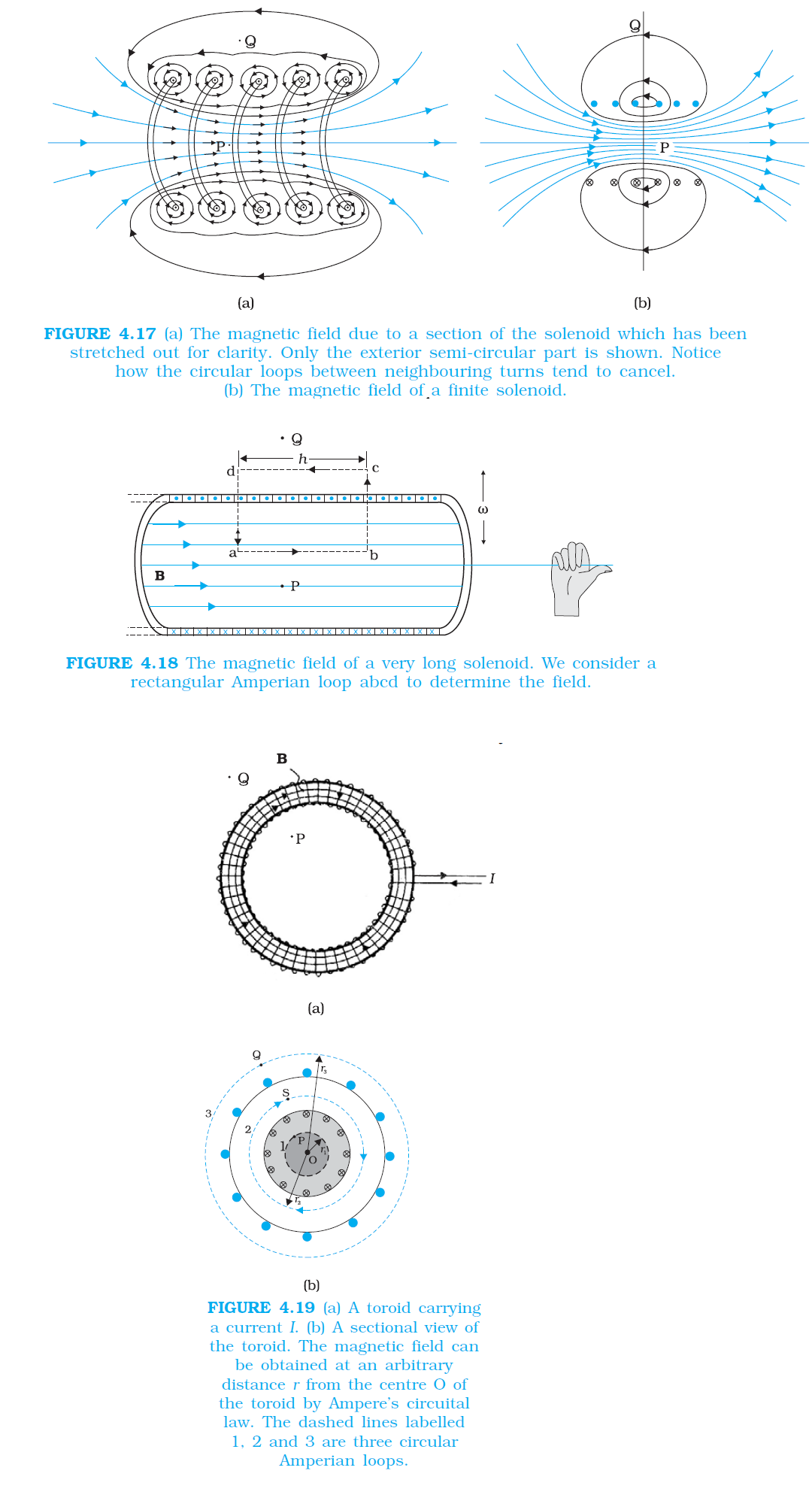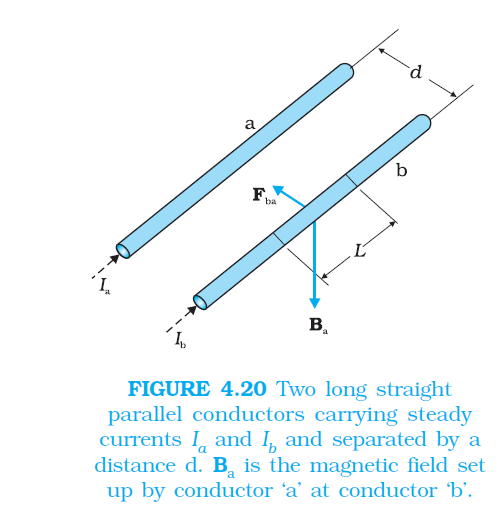Physics THE SOLENOID AND THE TOROID, FORCE BETWEEN TWO PARALLEL CURRENTS, THE AMPERE FOR CBSE-NCERT 3

### Topic covered

color{blue}{star} THE SOLENOID AND THE TOROID
color{blue}{star} FORCE BETWEEN TWO PARALLEL CURRENTS, THE AMPERE

### THE SOLENOIDcolor{blue} ✍️ The solenoid and the toroid are two pieces of equipment which generate magnetic fields. The television uses the solenoid to generate magnetic fields needed.

color{blue} ✍️ The synchrotron uses a combination of both to generate the high magnetic fields required. In both, solenoid and toroid, we come across a situation of high symmetry where Ampere’s law can be conveniently applied.

color{brown}bb ul("The solenoid")
color{blue} ✍️ We shall discuss a long solenoid. By long solenoid we mean that the solenoid’s length is large compared to its radius. It consists of a long wire wound in the form of a helix where the neighbouring turns are closely spaced.

color {blue}{➢➢} So each turn can be regarded as a circular loop. The net magnetic field is the vector sum of the fields due to all the turns. Enamelled wires are used for winding so that turns are insulated from each other.

color {blue}{➢➢}Figure 4.17 displays the magnetic field lines for a finite solenoid. We show a section of this solenoid in an enlarged manner in Fig. 4.17(a). Figure 4.17(b) shows the entire finite solenoid with its magnetic field. In Fig. 4.17(a), it is clear from the circular loops that the field between two neighbouring turns vanishes. In Fig. 4.17(b),

color{blue} ✍️ we see that the field at the interior mid-point P is uniform, strong and along the axis of the solenoid.

color {blue}{➢➢} The field at the exterior mid-point Q is weak and moreover is along the axis of the solenoid with no perpendicular or normal component. As the solenoid is made longer it appears like a long cylindrical metal sheet.

color{blue} ✍️ Figure 4.18 represents this idealised picture. The field outside the solenoid approaches zero. We shall assume that the field outside is zero. The field inside becomes everywhere parallel to the axis.color{blue} ✍️ Consider a rectangular Amperian loop abcd. Along cd the field is zero as argued above. Along transverse sections bc and ad, the field component is zero. Thus, these two sections make no contribution. Let the field along ab be B.

color {blue}{➢➢} Thus, the relevant length of the Amperian loop is, L = h. Let n be the number of turns per unit length, then the total number of turns is nh. The enclosed current is, I_e = I (n h), where I is the current in the solenoid. From Ampere’s circuital law [Eq. 4.17 (b)]

color{blue}(BL = mu_0I_e " ", Bh = mu_0 I(n h))

color{blue}(B = μ_0 n I)

...........(4.20)

color{blue} ✍️ The direction of the field is given by the right-hand rule. The solenoid is commonly used to obtain a uniform magnetic field. We shall see in the next chapter that a large field is possible by inserting a soft iron core inside the solenoid.
Q 3105601568A solenoid of length 0.5 m has a radius of 1 cm and is made up of 500 turns. It carries a current of 5 A. What is the magnitude of the magnetic field inside the solenoid?
Class 12 Chapter example 9Solution:

The number of turns per unit length is,

n = (500)/(0.5) = 1000 truns//m

The length l = 0.5 m and radius r = 0.01 m. Thus, l//a = 50 i.e., l > a.
Hence, we can use the long solenoid formula, namely, Eq. (4.20)

B = μ_0n I
= 4π × 10^(–7) × 10^3 × 5
= 6.28 × 10^(–3) T

### The toroidcolor{blue} ✍️ The toroid is a hollow circular ring on which a large number of turns of a wire are closely wound.

color {blue}{➢➢} It can be viewed as a solenoid which has been bent into a circular shape to close on itself. It is shown in Fig. 4.19(a) carrying a current I.

color{blue} ✍️ We shall see that the magnetic field in the open space inside (point P) and exterior to the toroid (point Q) is zero.

color{blue} ✍️ The field B inside the toroid is constant in magnitude for the ideal toroid of closely wound turns. Figure 4.19(b) shows a sectional view of the toroid. The direction of the magnetic field inside is clockwise as per the right-hand thumb rule for circular loops.

color{blue} ✍️ Three circular Amperian loops 1, 2 and 3 are shown by dashed lines. By symmetry, the magnetic field should be tangential to each of them and constant in magnitude for a given loop. The circular areas bounded by loops 2 and 3 both cut the toroid, so that each turn of current carrying wire is cut once by the loop 2 and twice by the loop 3.

color {blue}{➢➢} Let the magnetic field along loop 1 be B_1 in magnitude.

color {blue}{➢➢} Then in Ampere’s circuital law [Eq. 4.17(a)], color{navy}(L = 2π r_1.) However, the loop encloses no current, so color{navy}(I_e = 0.) Thus

color{blue}(B_1 (2pir_1) =mu_0 (0) B_1 = 0)

color {blue}{➢➢} Thus, the magnetic field at any point P in the open space inside the toroid is zero. We shall now show that magnetic field at Q is likewise zero. Let the magnetic field along loop 3 be B_3.

color {blue}{➢➢} Once again from Ampere’s law L = 2 π r_3. However, from the sectional cut, we see that the current coming out of the plane of the paper is cancelled exactly by the current going into it.

color {blue}{➢➢} Thus, I_e= 0, and B_3 = 0. Let the magnetic field inside the solenoid be B. We shall now consider the magnetic field at S. Once again we employ Ampere’s law in the form of Eq. [4.17 (a)]. We find, L = 2π r. The current enclosed I_e is (for N turns of toroidal coil) N I.

color{blue}(B (2πr) = μ_0NI)

color{blue}(B = (mu_0NI)/(2pir))

............(4.21)

color {blue}{➢➢} We shall now compare the two results: for a toroid and solenoid. We re-express Eq. (4.21) to make the comparison easier with the solenoid result given in Eq. (4.20).

color{blue} ✍️ Let r be the average radius of the toroid and be the number of turns per unit length.

Then color{blue}(N = 2πr n =) (average) perimeter of the toroid × number of turns per unit length

and thus,

color{blue}(B = μ_0 n I,)

...........(4.22)
i.e., the result for the solenoid In an ideal toroid the coils are circular. In reality the turns of the toroidal coil form a helix and there is always a small magnetic field external to the toroid.

### FORCE BETWEEN TWO PARALLEL CURRENTS, THE AMPERE

color{blue} ✍️ we have learnt that an external magnetic field will exert a force on a current-carrying conductor. This follows from the Lorentz force formula. Thus, it is logical to expect that two current-carrying conductors placed near each other will exert (magnetic) forces on each other. I

color {blue}{➢➢} Ampere studied the nature of this magnetic force and its dependence on the magnitude of the current, on the shape and size of the conductors as well as the distances between the conductors.

color{blue} ✍️ In this section, we shall take the simple example of two parallel current carrying conductors, which will perhaps help us to appreciate Ampere’s painstaking work. Figure 4.20 shows two long parallel conductors a and b separated by a distance d and carrying (parallel) currents I_a and I_b, respectively.

color {blue}{➢➢} The conductor ‘a’ produces, the same magnetic field B_a at all points along the conductor ‘b’. The right-hand rule tells us that the direction of this field is downwards (when the conductors are placed horizontally). Its magnitude is given by Eq. [4.19(a)] or from Ampere’s circuital law,

color{navy}(B_a = (mu_0I_a)/(2pid))

color{blue} ✍️ The conductor ‘b’ carrying a current I_b will experience a sideways force due to the field B_a. The direction of this force is towards the conductor ‘a’ (Verify this). We label this force as F_(ba), the force on a segment L of ‘b’ due to ‘a’. The magnitude of this force is given by Eq. (4.4),

color{navy}(F_(ba)= I_b LB_a)

..............(4.23)

color{navy}(= (mu_0I_aI_b)/(2pid)L)

color {blue}{➢➢} It is of course possible to compute the force on ‘a’ due to ‘b’. From considerations similar to above we can find the force F_(ab), on a segment of length L of ‘a’ due to the current in b. It is equal in magnitude to F_(ba), and directed towards ‘b’. Thus,

color{blue}(F_(ba)= - F_(ab))

.............(4.24)

color {brown}bbul{"Note that"} this is consistent with Newton’s third Law. Thus, at least for parallel conductors and steady currents, we have shown that the Biot-Savart law and the Lorentz force yield results in accordance with Newton’s third Law*.

color {blue}{➢➢} We have seen from above that currents flowing in the same direction attract each other. One can show that oppositely directed currents repel each other. Thus, Parallel currents attract, and antiparallel currents repel.

color {blue}{➢➢} This rule is the opposite of what we find in electrostatics. Like (same sign) charges repel each other, but like (parallel) currents attract each other.

color{blue} ✍️ Let color{blue}(f_(ba)) represent the magnitude of the force F_(ba) per unit length. Then, from Eq. (4.23),

color{blue}(f_(ba) = (mu_0I_aI_b)/(2pid))

................(4.25)

color {blue}{➢➢} The above expression is used to define the ampere (A), which is one of the seven SI base units.

color {blue}{➢➢} The ampere is the value of that steady current which, when maintained in each of the two very long, straight, parallel conductors of negligible cross-section, and placed one metre apart in vacuum, would produce on each of these conductors a force equal to 2 × 10^(–7) newtons per metre of length.

color {blue}{➢➢} In practice one must eliminate the effect of the earth’s magnetic field and substitute very long wires by multiturn coils of appropriate geometries. An instrument called the current balance is used to measure this mechanical force.

color {blue}{➢➢} The SI unit of charge, namely, the coulomb, can now be defined in terms of the ampere. When a steady current of 1A is set up in a conductor, the quantity of charge that flows through its cross-section in 1s is one coulomb (1C).